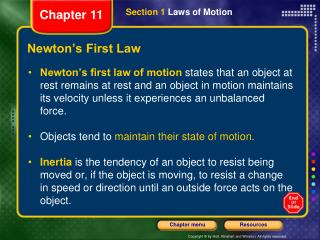DownloadDownload PresentationNewton’s First Law

# Newton’s First Law

Download Presentation## Newton’s First Law

- - - - - - - - - - - - - - - - - - - - - - - - - - - E N D - - - - - - - - - - - - - - - - - - - - - - - - - - -
##### Presentation Transcript

1. Section 1 Laws of Motion Chapter 11 Newton’s First Law • Newton’s first law of motionstates thatan object at rest remains at rest and an object in motion maintains its velocity unless it experiences an unbalanced force. • Objects tend to maintain their state of motion. • Inertiais the tendency of an object to resist being moved or, if the object is moving, to resist a change in speed or direction until an outside force acts on the object.

2. Section 1 Laws of Motion Chapter 11 Newton’s First Law

3. Section 1 Laws of Motion Chapter 11 Newton’s First Law, continued • Inertia is related to an object’s mass. Mass is a measure of inertia. • Seat belts and car seats provide protection. • Because of inertia, you slide toward the side of a car when the driver makes a sharp turn. • When the car you are riding in comes to a stop, your seat belt and the friction between you and the seat stop your forward motion.

4. Section 1 Laws of Motion Chapter 11 Mass and Inertia

5. Section 1 Laws of Motion Chapter 11 Newton’s Second Law • Newton’s second law of motionstates that the unbalanced force acting on an object equals the object’s mass times its acceleration. • Force equals mass times acceleration. Force = mass  acceleration F = ma • Force is measured in newtons (N). 1 N = 1 kg  1 m/s2

6. Section 1 Laws of Motion Chapter 11 Newton’s Second Law

7. Section 1 Laws of Motion Chapter 11 Newton’s Second Law

8. Section 1 Laws of Motion Chapter 11 Math Skills Newton’s Second Law Zookeepers lift a stretcher that holds a sedated lion. The total mass of the lion and stretcher is 175 kg, and the lion’s upward acceleration is 0.657 m/s2. What is the unbalanced force necessary to produce this acceleration of the lion and the stretcher? 1. List the given and unknown values. Given: mass, m = 175 kg acceleration, a = 0.657 m/s2 Unknown: force, F = ? N

9. Section 1 Laws of Motion Chapter 11 Math Skills, continued 2. Write the equation for Newton’s second law. force = mass  acceleration F = ma 3. Insert the known values into the equation, and solve. F = 175 kg  0.657 m/s2 F = 115 kg m/s2 = 115 N

10. Section 1 Laws of Motion Chapter 11 Newton’s Second Law, continued • Newton’s second law can also be stated as follows: The acceleration of an object is proportional to the net force on the object and inversely proportional to the object’s mass.